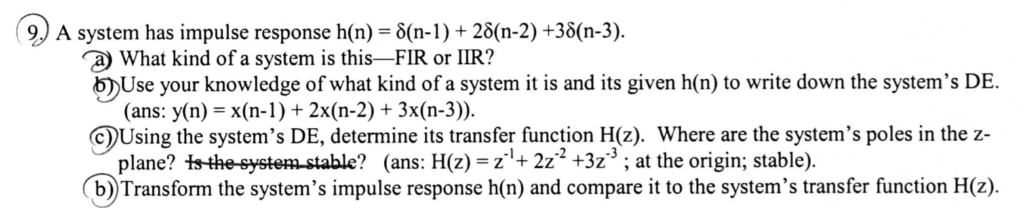### Create an Account

Home / Questions / 9J A system has impulse response h(n) = 8(n-1) + 28(n-2) +38(n-3) a What kind of a system ...

# 9J A system has impulse response h(n) = 8(n-1) + 28(n-2) +38(n-3) a What kind of a system is this-FI9J A system has impulse response h(n) = 8(n-1) + 28(n-2) +38(n-3) a What kind of a system is this-FIR or IIR? bUse your knowledge of what kind of a system it is and its given h(n) to write down the system&#39;s DE. (ans: y(n) x(n-1) + 2x(n-2) +3x(n-3)) CUsing the system&#39;s DE, determine its transfer function H(z). Where are the system&#39;s poles in the z- plane? tsthe-system stable? (ans: H(z) = z+2z2 +3z3 ; at the origin; stable) b) Transform the system&#39;s impulse response h(n) and compare it to the system&#39;s transfer function H(z)

Apr 01 2020 View more View LessSubscribe To Get Solution